Courses

# Summing Amplifier : Applications of Op-amp - CSIR-NET Physical Sciences Physics Notes | EduRev

## Physics for IIT JAM, UGC - NET, CSIR NET

Created by: Akhilesh Thakur

## Physics : Summing Amplifier : Applications of Op-amp - CSIR-NET Physical Sciences Physics Notes | EduRev

The document Summing Amplifier : Applications of Op-amp - CSIR-NET Physical Sciences Physics Notes | EduRev is a part of the Physics Course Physics for IIT JAM, UGC - NET, CSIR NET.
All you need of Physics at this link: Physics

The Summing Amplifier is another type of operational amplifier circuit configuration that is used to combine the voltages present on two or more inputs into a single output voltage.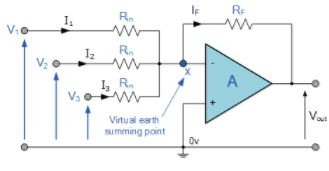We saw previously in the inverting operational amplifier that the inverting amplifier has a single input voltage, (Vin) applied to the inverting input terminal. If we add more input resistors to the input, each equal in value to the original input resistor, (Rin) we end up with another operational amplifier circuit called a Summing Amplifier, “summing inverter” or even a “voltage adder” circuit as shown below.

Summing Amplifier Circuit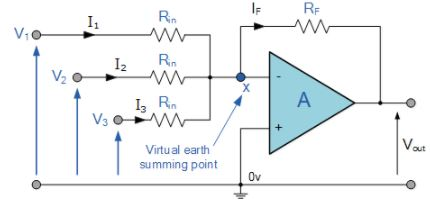In this simple summing amplifier circuit, the output voltage, ( Vout ) now becomes proportional to the sum of the input voltages, V1, V2, V3, etc. Then we can modify the original equation for the inverting amplifier to take account of these new inputs thus: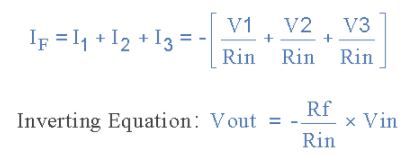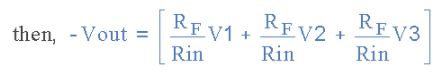However, if all the input impedances, ( Rin ) are equal in value, we can simplify the above equation to give an output voltage of:

Summing Amplifier EquationWe now have an operational amplifier circuit that will amplify each individual input voltage and produce an output voltage signal that is proportional to the algebraic “SUM” of the three individual input voltages V1, V2 and V3. We can also add more inputs if required as each individual input “see’s” their respective resistance, Rin as the only input impedance.

This is because the input signals are effectively isolated from each other by the “virtual earth” node at the inverting input of the op-amp. A direct voltage addition can also be obtained when all the resistances are of equal value and Rƒ is equal to Rin.

Note that when the summing point is connected to the inverting input of the op-amp the circuit will produce the negative sum of any number of input voltages. Likewise, when the summing point is connected to the non-inverting input of the op-amp, it will produce the positive sum of the input voltages.

Scaling Summing Amplifier can be made if the individual input resistors are “NOT” equal. Then the equation would have to be modified to:To make the math’s a little easier, we can rearrange the above formula to make the feedback resistor Rƒ the subject of the equation giving the output voltage as:This allows the output voltage to be easily calculated if more input resistors are connected to the amplifiers inverting input terminal. The input impedance of each individual channel is the value of their respective input resistors, ie, R1, R2, R3 … etc.

Sometimes we need a summing circuit to just add together two or more voltage signals without any amplification. By putting all of the resistances of the circuit above to the same value R, the op-amp will have a voltage gain of unity and an output voltage equal to the direct sum of all the input voltages as shown: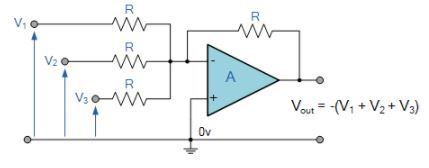The Summing Amplifier is a very flexible circuit indeed, enabling us to effectively “Add” or “Sum” (hence its name) together several individual input signals. If the inputs resistors, R1, R2, R3 etc, are all equal a “unity gain inverting adder” will be made. However, if the input resistors are of different values a “scaling summing amplifier” is produced which will output a weighted sum of the input signals.

Summing Amplifier Example No1

Find the output voltage of the following Summing Amplifier circuit.

Summing Amplifier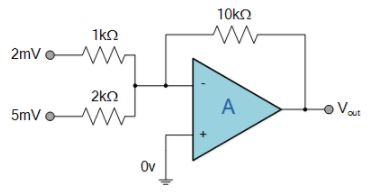Using the previously found formula for the gain of the circuit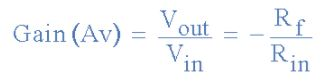We can now substitute the values of the resistors in the circuit as follows,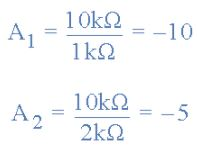We know that the output voltage is the sum of the two amplified input signals and is calculated as: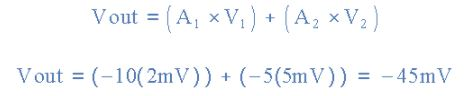Then the output voltage of the Summing Amplifier circuit above is given as -45 mV and is negative as its an inverting amplifier.

Summing Amplifier Applications

So what can we use summing amplifiers for? If the input resistances of a summing amplifier are connected to potentiometers the individual input signals can be mixed together by varying amounts.

For example, measuring temperature, you could add a negative offset voltage to make the output voltage or display read “0” at the freezing point or produce an audio mixer for adding or mixing together individual waveforms (sounds) from different source channels (vocals, instruments, etc) before sending them combined to an audio amplifier.

Summing Amplifier Audio Mixer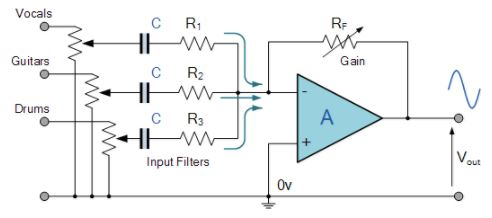Another useful application of a Summing Amplifier is as a weighted sum digital-to-analogue converter. If the input resistors, Rin of the summing amplifier double in value for each input, for example, 1kΩ, 2kΩ, 4kΩ, 8kΩ, 16kΩ, etc, then a digital logical voltage, either a logic level “0” or a logic level “1” on these inputs will produce an output which is the weighted sum of the digital inputs. Consider the circuit below.

Digital to Analogue Converter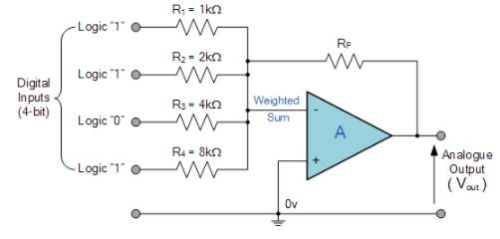Of course this is a simple example. In this DAC summing amplifier circuit, the number of individual bits that make up the input data word, and in this example 4-bits, will ultimately determine the output step voltage as a percentage of the full-scale analogue output voltage.

Also, the accuracy of this full-scale analogue output depends on voltage levels of the input bits being consistently 0V for “0” and consistently 5V for “1” as well as the accuracy of the resistance values used for the input resistors, Rin.

Fortunately to overcome these errors, at least on our part, commercially available Digital-to Analogue and Analogue-to Digital devices are readily available with highly accurate resistor ladder networks already built-in.

159 docs

,

,

,

,

,

,

,

,

,

,

,

,

,

,

,

,

,

,

,

,

,

;